Next: Formula to compute compound Up: Formulas of General Interest Previous: Symbolic Computation Packages

# Formula for the Surface Area of a sphere in Euclidean N-Space

This is equivalent to the volume of the N-1 solid which comprises the boundary of an N-Sphere.

The volume of a ball is the easiest formula to remember: It's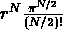. The only hard part is taking the factorial of a half-integer. The real definition is that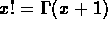, but if you want a formula, it's: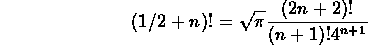To get the surface area, you just differentiate to get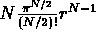.

There is a clever way to obtain this formula using Gaussian integrals. First, we note that the integral over the line of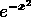is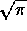. Therefore the integral over N-space of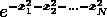is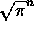. Now we change to spherical coordinates. We get the integral from 0 to infinity of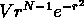, where V is the surface volume of a sphere. Integrate by parts repeatedly to get the desired formula.

It is possible to derive the volume of the sphere from ``first principles''.

Alex Lopez-Ortiz
Mon Feb 23 16:26:48 EST 1998